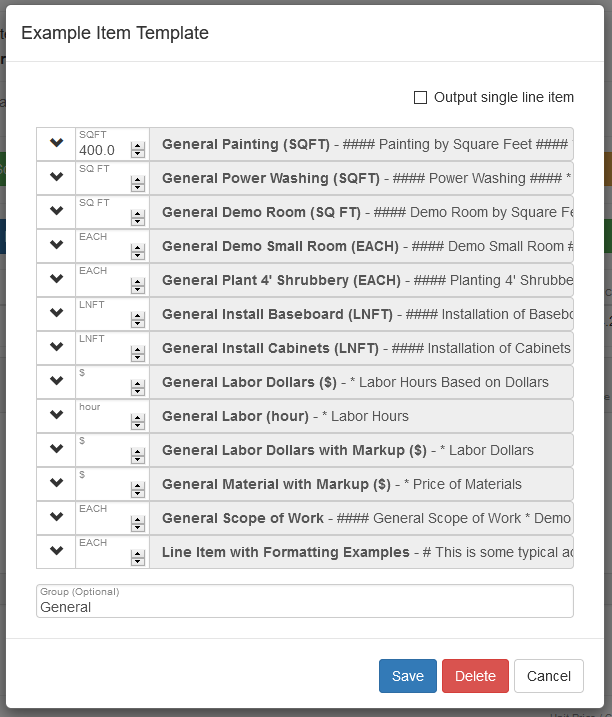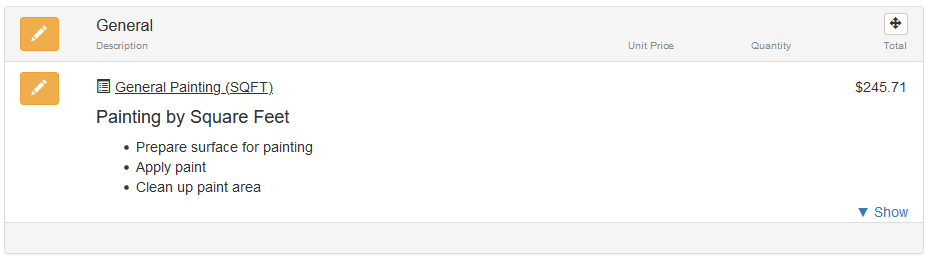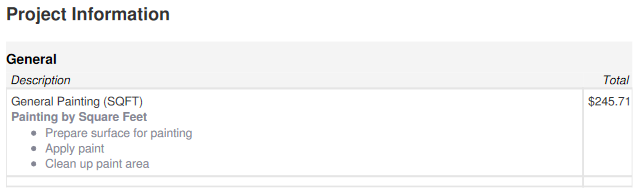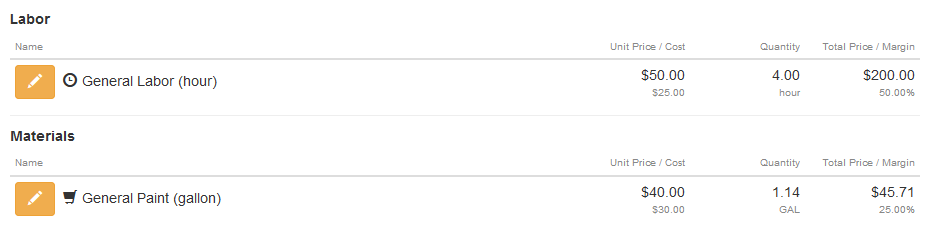# General Painting (square-feet) - Used in Project

The general painting (square feet) item assembly is based on a production rate of 100 square feet per hour and a coverage rate of the paint at 350 square feet per gallon. The Estimate Rocket smart line items output 3 sets of information when an item is added to a project:

1. Price and detailed work description for your customer to see
2. Estimated profit margin calculation
3. Time and materials calculations for work orders

Click here to see how to modify the general painting (square feet) for specific use.

In this sample project area is going to have general painting work done

Line Item is Added Directly to the Project• Painting measurement = 400 square feet
• Production rate of installation = 100 square feet per hour
• Labor Calculation 1 = 400 square feet / 100 square feet per hour = 4 hours needed
• Labor Calculation 2 = 4 hours x \$50 per hour = \$200 labor dollars
• Material Calculation 1 = 400 square feet / 350 square feet per gallon = 1.14 gallon of material needed
• Material Calculation 2 = 1.14 gallon x \$40 per gallon = \$45.71 material dollars
• Total Calculation \$200 labor dollars + \$45.71 material dollars = \$245.71 total dollarsThe square footage painting is priced at \$245.71 and the customer will see that price on their estimate along with a detailed description of the work to be done.

A Preview of the Estimate shows the customer the price and detailed descriptionEstimated Profit Margin

The general painting also calculates an estimated profit margin for this project as seen in the project detail screen.In this case there are labor and material components involved in the assembly, the price to the customer is \$0.61 per square foot and the cost is \$0.34 per square foot. The profit margin for the general painting is 45.35% based on the default item setup.The labor and materials are also calculated for in the work order. The labor and materials section is not displayed on the customer estimate but is displayed in the work order.The labor time and material needed are both calculated based on this assembly.

Click here to see how to modify the general painting (square feet) for specific use.

Back to the Example Item Template

All Available Item Templates